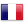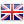﻿ Test the PHP function substr

# Test the PHP function substr

This tool will allow you to test the PHP substr function without coding. Simply enter the start and length (optional) settings.

`substr` is a php function which extracts a portion of a string.

This function takes three parameters: a string, a start integer and an optional integer for the length.

Settings

- Data
It must have a length of at least 1 character.

- Start
• If start is positive, the returned string will start at the position of 'start' in the string, counting from zero. For example, in the string 'abcdef', the character at position 0 is 'a', the character at position 2 is 'c', and so on.
• If start is negative, the returned string will start with the character at the position 'start' from the end of the string.
• If start is greater than the length of the string, FALSE will be returned.

- Length
• If length is provided and positive, the returned string will contain at most the length of characters beginning from the start (depending on the length of the string).
• If length is provided and negative, then this number of characters will be omitted from the end of the string (after the starting position has been calculated when beginning is negative). If start indicates an starting truncation after the start indicated by length, FALSE is returned.
• If length is given and equal to 0, FALSE or NULL, an empty string is returned.
• If length is omitted, the string from the beginning to the end of the string is returned.

``````<?php
\$rest = substr("abcdef", 0, -2);  // returns "abcd"
\$rest = substr("abcdef", 2, -1);  // returns "cde"
\$rest = substr("abcdef", 4, -3);  // returns false
\$rest = substr("abcdef", -3, -1); // returns "de"
\$rest = substr("abcdef", 3); // returns "def"
?>
``````FREN